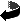MATH 554 - Math 703 I
Analysis I

Homework Assignments and Supplementary Materials

To view an Acrobat PDF version of each Solution Set, click on the symbol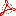.
(Free Acrobat reader can be found here.)
The icon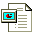indicates a PowerPoint file.

Homework Assignments
(Each Problem is worth 10 pts.)

 No. Assignment Due Page and No.'s Solutions #1 Aug. 30 (Thur.) Assignment #1#2 Sept. 6 (Thur.) Assignment #2#3 Sept. 13 (Thur.) Prove that the product of a nonzero rational with an irrational is irrational. Page 28: 8, 10, 20#4 Sept. 18 (Tues.) Page 35: 1 a),d), and (f) ; 9 Prove that If 1 < a and 1 < b, then 1 < ab. If 0 < a and n is a natural number, then 1 < (1+a)n. If 0 < a and n is a natural number, then 1 + na < (1+a)n.#5 Oct. 9 (Tues.) Page 58-60: 6, 9 b), 11, 28;   Page 66: 2 Extra Credit: Page 59: 17;   Page 66: 3#6 Oct. 18 (Thur.) Prove each of the following: Both IR and Æ are closed sets. Arbitrary intersections of closed sets are closed. Finite unions of closed sets are closed. {0,1,[1/2],[1/3], ..., [1/n], ... } is a closed set. In each problem justify your answer. The "space" in each case is IR. Find the set of limit points for the set {1,1/2,1/3, ...}. Determine the set of limit points for the set of rational numbers in the interval (0,1]. Determine the limit points for the set [-1,2) È {5/2} È [5,6].#7 Nov. 6 (Tues.) Assignment #7 #8 Nov. 13 (Tues.) Assignment #8 #9 Nov. 20 (Tues.) Assignment #9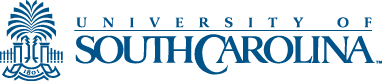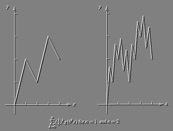This page maintained by Robert Sharpley (sharpley@math.sc.edu) and last updated Aug. 28, 2001.  This page ©2000-2001, The Board of Trustees of the University of South Carolina.  URL: http://www.,math.sc.edu/~sharpley/math554

Return to Math 554 Home Page